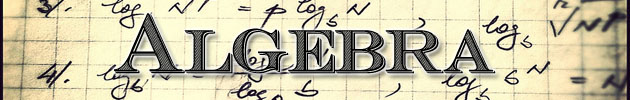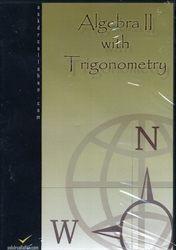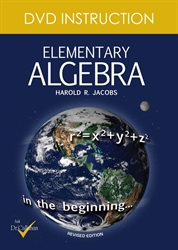#Together with geometry, analysis, and number theory, algebra is one of the several main branches of mathematics. Elementary algebra is usually part of the curriculum in early high school and provides an introduction to the basic ideas of algebra, including effects of adding and multiplying numbers, the concept of variable, definition of polynomials, along with factorization and determining their roots. However, algebra is much broader than this. In addition to working directly with numbers, algebra covers working with symbols, variables, and set elements. Addition and multiplication are viewed as general operations, and their precise definitions lead to structures such as groups, rings and fields.

Most students at the high school level are being exposed only to elementary algebra—its most basic form. It is taught to students who are presumed to have no knowledge of mathematics beyond the basic principles of arithmetic. In arithmetic, only numbers and their arithmetical operations (such as +, -, ×, ÷) occur; in algebra, numbers are often denoted by symbols (such as a, x, y). This is useful because:

• It allows the general formulation of arithmetical laws (such as a + b = b + a for all a and b), and thus is the first step to a systematic exploration of the properties of the real number system.
• It allows the reference to "unknown" numbers, the formulation of equations and the study of how to solve these (for instance, Find a number x such that 3x + 1 = 10").
• It allows the formulation of functional relationships (such as "If you sell x tickets, then your profit will be 3x - 10 dollars, or f(x) = 3x - 10, where f is the function, and x is the number the function is performed on.").Algebra 2 with Trigonometry - DVD from AskDrCallahan for 10th-12th grade in Trigonometry (Location: MATR-TRIG) \$145.00Elementary Algebra - DVD by Cassidy Cash from AskDrCallahan for 8th-10th grade in Harold Jacobs Mathematics (Location: MAT-MB3) \$48.00 (2 in stock)Elementary Algebra - DVD by Cassidy Cash from Master Books for 8th-10th grade in Harold Jacobs Mathematics (Location: MAT-MB3) \$95.99